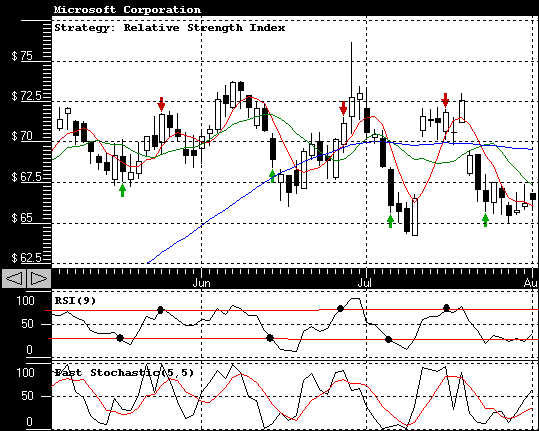## Stock Predictor - Relative Strength Index

This strategy is based on an absolute values of Relative Strength Index (RSI). Typically when RSI reaches level of 80 it is considered that a security is overbought and when RSI is hitting 20 it is considered that a security is oversold. The current strategy does the following : it generates a buy signal when RSI is falling below a set limit a (a=30 in the example) and is generating a sell signal when RSI reaches a sell limit b (b=70 in the example)

<< Back to the Stock Predictor